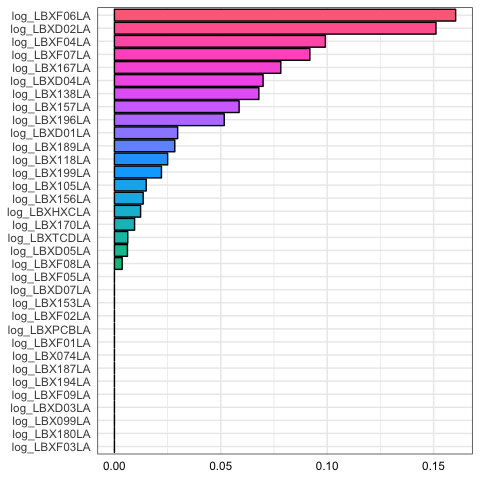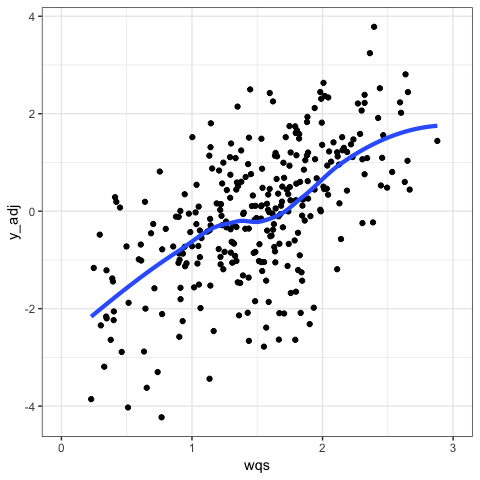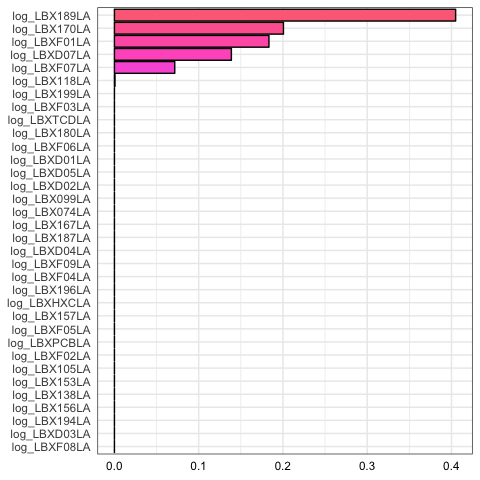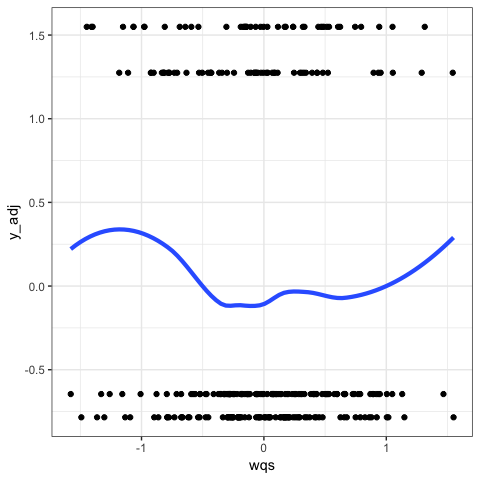# How to use gWQS package

## Introduction

Weighted Quantile Sum (WQS) regression is a statistical model for multivariate regression in high-dimensional datasets commonly encountered in environmental exposures, epi/genomics, and metabolomic studies, among others. The model constructs a weighted index estimating the mixed effect of all predictor variables on an outcome, which may then be used in a regression model with relevant covariates to test the association of the index with a dependent variable or outcome. The contribution of each individual predictor to the overall index effect may then be assessed by the relative strength of the weights the model assigns to each variable.

The gWQS package extends WQS regression to applications with continuous and categorical outcomes. In practical terms, the primary outputs of an analysis will be the parameter estimates and significance tests for the overall index effect of predictor variables, and the estimated weights assigned to each predictor, which identify the relevant contribution of each variable to the relationship between the WQS index and the outcome variable.

For additional theoretical background on WQS regression, see the references provided below.

## How to use the gWQS package

### Example 1

The main function of the gWQS package is gwqs, which allows the implementation of Weighted Quantile Sum Regression for linear and a logistic regression. We created the wqs_data dataset (available once the package is installed and loaded) to show how to use this function. These data reflect 34 exposure concentrations simulated from a distribution of PCB exposures measured in subjects participating in the NHANES study (2001-2002). Additionally, an end-point meaure, simulated from a distribution of leukocyte telomere length (LTL), a biomarker of chronic disease, is provided as well (variable name: y), as well as simulated covariates, e.g. sex, and a dichotomous outcome variable (variable name: disease_state). This dataset can thus be used to test the gWQS package by analyzing the mixed effect of the 34 simulated PCBs on the continuous or binary outcomes, with adjustments for covariates.

Here is an overview of the dataset:

y disease_state sex log_LBX074LA log_LBX099LA log_LBX105LA log_LBX118LA log_LBX138LA log_LBX153LA log_LBX156LA log_LBX157LA log_LBX167LA log_LBX170LA log_LBX180LA log_LBX187LA log_LBX189LA log_LBX194LA log_LBX196LA log_LBX199LA log_LBXD01LA log_LBXD02LA log_LBXD03LA log_LBXD04LA log_LBXD05LA log_LBXD07LA log_LBXF01LA log_LBXF02LA log_LBXF03LA log_LBXF04LA log_LBXF05LA log_LBXF06LA log_LBXF07LA log_LBXF08LA log_LBXF09LA log_LBXPCBLA log_LBXTCDLA log_LBXHXCLA
-0.4224332 1 0 0.0680716 -0.1608614 0.9648013 -0.1815684 0.7506797 0.9363278 -0.0364088 0.1971499 1.1192873 0.2972489 0.1920384 -0.5257044 -0.1659253 0.4017490 -0.0143133 0.8692975 0.0846309 0.0012511 -0.2847784 0.6327454 0.4078422 0.1661874 -1.2905580 -1.0359049 1.6893966 -0.1942655 0.4322813 -1.8049708 -0.7816081 0.2459645 -1.0917383 -0.3476487 -0.0358878 1.2711118
0.7613784 0 0 0.5276676 1.4403102 0.7753093 0.1055764 -0.9015101 0.1677693 0.7643587 -0.6001477 0.6175614 -0.3577213 0.2879546 -0.1557095 -0.6755189 0.0519114 0.3351334 -1.1385368 0.5852100 -1.4539998 1.1116691 0.0767604 -0.0295292 -1.2578515 -0.0246641 0.5600564 0.1143720 1.1365974 0.8777775 -0.1214907 0.7902525 0.2255480 -1.0510728 0.5891812 -0.6304355 0.7265865
-2.1113324 1 1 -1.8650139 1.4885628 -1.5420050 -0.5412270 -1.3624492 -0.4177779 0.1414001 0.1333590 -0.9901708 0.1024100 -0.0622932 1.0824292 -0.6920183 -1.7204760 -1.2079225 0.3543356 -0.8116185 -0.8790250 0.4616429 -2.0101411 -1.2859585 0.8281122 0.0921212 -0.6980090 0.2987547 -0.0450820 0.4917081 0.3754392 -1.6193861 0.0812163 0.2236327 -0.5211774 -1.0174183 -0.4092153
2.2222394 0 0 1.2585209 1.6110700 2.2069536 1.3286183 1.8871201 1.8393058 2.2751535 1.1307767 1.2204913 0.1958512 0.9461497 -0.0325942 -0.3940764 0.2984753 0.7837346 1.9352485 0.1973576 0.8376586 -1.2715081 -1.2577145 -0.5786619 0.6304414 1.3148279 0.2485757 -0.4723713 0.7557946 0.3307601 -0.0342781 -0.2875164 0.4176680 0.0977815 -1.1273511 1.0095760 0.6639172
-0.2190805 1 0 -1.0138713 -1.9903436 -0.6009229 -0.8907467 -0.4789443 -2.8190387 -0.0095402 -1.5477137 0.5180853 -2.1491031 -2.7951863 -1.5755779 -0.5270997 -0.2450904 -1.1242993 -0.6902691 -0.5791061 -1.9495504 -1.3426006 0.6178038 -1.2587666 -0.3310535 -1.5882632 -1.3967154 -1.2128921 -0.8581706 -0.6420270 -1.6946821 -0.2143440 -1.4720725 -0.5997930 -1.0896972 -0.3474791 -0.7175427
-1.7282770 0 0 -0.9752573 -0.6864727 0.3389021 0.5984246 0.0270300 -0.4798327 -0.3867123 -1.0377681 -0.7329465 -0.7148301 0.4060471 -0.5076881 0.2215813 0.3212146 -0.1809994 1.4622912 0.0165252 -0.6026058 -0.0865081 -0.7574191 -0.0918236 -0.3804055 -0.0150627 0.5944020 0.4030363 -0.1233755 -0.7816779 -0.3795485 0.4316160 1.7915524 -0.9154308 0.2882417 0.6230951 1.4571064
0.4219639 0 1 -0.6225983 1.0113598 -0.2162429 0.7575008 -0.2571729 0.9804511 0.4458043 1.3962681 -0.1979610 0.1995122 1.4669089 1.0634414 0.8339600 1.5867141 0.7249490 0.7584681 0.2701363 0.9317193 0.0963273 0.3944993 0.6827900 -0.5437556 0.9504820 0.5142011 0.5474847 0.4452620 -0.4822166 0.7318831 0.0323481 -0.9661976 0.7297474 -0.1620114 0.5754746 0.9274046
-0.4762419 0 1 -0.1801051 -0.5805092 -0.8038376 -0.7786034 -1.5457539 -1.3117169 -0.9734300 -0.0261900 0.5308421 -0.2485865 0.2103118 -0.0912344 -0.1011264 -0.4217051 0.4682131 -0.9008740 -0.4390236 -1.0384994 -1.0510064 -0.1617107 -1.5014766 -1.1384429 1.7696655 1.9844850 -0.4584083 -1.8996102 -1.0736679 1.2719959 1.2918048 -0.0684242 0.4754351 -1.0453685 0.0445513 -1.8944198
-0.5126990 1 0 0.4502187 0.7892304 0.7107290 0.2754549 0.0613806 0.3399312 1.0176518 1.1807174 1.2440091 1.5589123 -0.8405278 -0.2002233 0.2201809 0.2284441 -0.1742271 -0.1887278 0.3027553 -0.3797491 -0.4089264 -0.5428011 1.2964970 -0.2374201 0.1206623 0.4179228 1.3594435 1.6920543 -0.3431321 -0.2684203 0.9664860 -0.0108648 -0.9556556 2.0269212 0.0600171 0.4541168
-0.8479847 1 0 -0.4461221 -1.0556249 1.0676569 0.0681564 -0.9582167 -0.3967903 0.0196100 0.0467119 0.5172333 0.2781621 0.1674821 1.1879319 -1.0221745 -1.3879976 -0.7295127 -1.7526492 -0.7668207 0.5923798 1.0463819 0.9856947 -1.1673793 -0.7855519 -0.8638427 -0.7379376 -0.0984674 -0.7446359 -0.2253229 -0.9085362 -0.8710364 1.6918778 -0.8597897 -0.4343029 0.4885121 -1.5592026

WQS with a continuous outcome: This script calls a wqs model for a continuous outcome using the function gwqs.

# we save the names of the mixture variables in the variable "mix_name"
toxic_chems = c("log_LBX074LA", "log_LBX099LA", "log_LBX105LA", "log_LBX118LA",
"log_LBX138LA", "log_LBX153LA", "log_LBX156LA", "log_LBX157LA",
"log_LBX167LA", "log_LBX170LA", "log_LBX180LA", "log_LBX187LA",
"log_LBX189LA", "log_LBX194LA", "log_LBX196LA", "log_LBX199LA",
"log_LBXD01LA", "log_LBXD02LA", "log_LBXD03LA", "log_LBXD04LA",
"log_LBXD05LA", "log_LBXD07LA", "log_LBXF01LA", "log_LBXF02LA",
"log_LBXF03LA", "log_LBXF04LA", "log_LBXF05LA", "log_LBXF06LA",
"log_LBXF07LA", "log_LBXF08LA", "log_LBXF09LA", "log_LBXPCBLA",
"log_LBXTCDLA", "log_LBXHXCLA")

# we run the model and save the results in the variable "results"
results = gwqs(y ~ NULL, mix_name = toxic_chems, data = wqs_data, q = 4, validation = 0.6,
valid_var = NULL, b = 3, b1_pos = T, b1_constr = F, family = "gaussian",
seed = 2016, wqs2 = T, plots = T, tables = T)

 “The optimization function did not converge 0 times”This WQS model tests the relationship between our dependent variable, y, and a WQS index estimated from ranking exposure concentrations in quartiles (q = 4). It also divided the data for training and validation, with 40% of the dataset for training and 60% for validation (validation = 0.6), and assigned 3 bootstrapping steps (b = 3) for parameter estimation. We chose to let the function create the training and validation dataset by itself (valid_var = NULL). Because WQS provides an unidirectional evaluation of mixture effects, we first examined weights derived from bootstrapped models where $$\beta_1$$ was positive (b1_pos = T); we could test for negative associations by testig that parameter to false (b1_pos = F). We can also choose to constraint the $$\beta_1$$ to be positive (b1_pos = T and b1_constr = T) or negative (b1_pos = F and b1_constr = T) when we estimate the weights; in this case we are not applying a constraint to $$\beta_1$$. We linked our model to a gaussian distribution to test for relationships between the continuous outcome and exposures (family = "gaussian"), and fixed the seed to 2016 for reproducible results (seed = 2016). Since we suspected a non-linear dynamic we added the wqs2 parameter (wqs2 = T) to include a quadratic term in the model. We plotted both a summary model with loess fit and a summary of each variables relative weight (plots = T). Finally, in the directory we saved summaries (tables = T) for the linear (Summary_results.html) and the quadratic model (Summary_results_quadratic.html), the results of ANOVA (Aov_results.html) and the table with the weights (Weights_table.html). A table with the regression results is printed automatically in the Viewer Pane.

The first plot is a barplot showing the weights assigned to each variable ordered from the highest weight to the lowest. These results indicate that the variables log_LBXF06LA, log_LBXD02LA, and log_LBXF04LA are the largest contributors to this mixture effect, with the first 7 chemicals explaining over the 70% of the total weights. The same information is contained in the table results$final_weights: mix_name mean_weight log_LBXF06LA 0.160 log_LBXD02LA 0.151 log_LBXF04LA 0.099 log_LBXF07LA 0.092 log_LBX167LA 0.078 log_LBXD04LA 0.070 log_LBX138LA 0.068 log_LBX157LA 0.059 log_LBX196LA 0.052 log_LBXD01LA 0.030 log_LBX189LA 0.028 log_LBX118LA 0.025 log_LBX199LA 0.022 log_LBX105LA 0.015 log_LBX156LA 0.014 log_LBXHXCLA 0.012 log_LBX170LA 0.009 log_LBXTCDLA 0.006 log_LBXD05LA 0.006 log_LBXF08LA 0.004 log_LBXF05LA 0.000 log_LBXD07LA 0.000 log_LBX153LA 0.000 log_LBXF02LA 0.000 log_LBXPCBLA 0.000 log_LBXF01LA 0.000 log_LBX074LA 0.000 log_LBX187LA 0.000 log_LBX194LA 0.000 log_LBXF09LA 0.000 log_LBXD03LA 0.000 log_LBX099LA 0.000 log_LBX180LA 0.000 log_LBXF03LA 0.000 In the second plot we have a representation of the wqs index vs the outcome (adjusted for the model residual when covariates are included in the model) that show the direction and the shape of the association between the exposure and the outcome. For example in this case we can observe a linear and positive relationship between the mixture and the y variable. To test the statistical significance of the association between the variables in the model, the following code has to be run: summary(results$fit)

These are the results for our example:

Fitting linear model: y ~ .
Estimate Std. Error t value Pr(>|t|)
(Intercept) -2.304 0.1875 -12.29 2.394e-28
wqs 1.492 0.1176 12.69 8.729e-30

This last table tells us that the association is positive and statistically significant (p<0.001).

Since we decided to add also the wqs quadratic term (wqs2 = TRUE), the gwqs function fits a second model adding the wqs quadratic term. The code to view the results of the test is the following:

summary(results$fit_2) Fitting linear model: y ~ . Estimate Std. Error t value Pr(>|t|) (Intercept) -2.212 0.356 -6.214 1.741e-09 wqs 1.345 0.5 2.689 0.007574 wqs_2 0.0508 0.167 0.3042 0.7612 The quadratic term is not significant in our model confirming that the relationship between the outcome and the exposure is linear (as shown by the previous plot). There is also the option to compare the two models with the analysis of variance: results$aov
Analysis of Variance Table
Res.Df RSS Df Sum of Sq Pr(>Chi)
298 376.9 NA NA NA
297 376.7 1 0.1174 0.761

Where the first line refers to the simple model and the second to the model with the wqs quadratic term. The results confirm that the linear model best explains the relationship between the outcome and the exposure.

The gwqs function gives back other outputs like the vector of the estimated $$\beta_1$$ in each bootstrap sample (results$b1), the vector of the values that indicate whether the solver has converged (0) or not (1 or 2) (results$conv), the matrix with all the estimated weights, $$\beta_1$$ and p-values for each bootstrap sample (results$wb1pm), the vector containing the y values adjusted for the residuals of the fitted model when it is covariates adjusted (results$y_adj), the list of vectors containing the rownames of the subjects included in each bootstrap dataset (results$index_b), the data frame containing the subjects used to estimate the weights in each bootstrap (results$data_t), the data frame containing the subjects used to estimate the parameters of the final model (results$data_v) and the data frame containing the final weights associated to each chemical (results$final_weights).

### Example 2

In the following code we run a logistic regression (family = "binomial") to test the association between the exposure to the mixture and the outcome disease_state and we also add the covariate sex. Since the mixture concentrations are already standardized we can also run a model without categorizing for quantiles (q = NULL). We chose to create the training and validation dataset and assign to valid_var the name of the variable that identifies the two datasets (valid_var = "group"). Furthermore we examined weights derived from bootstrapped models where $$\beta_1$$ was negative (b1_pos = F) since (as we can see in the following plot) there is a negative association between the exposure and the outcome:

# we create the variable "group" in the dataset to identify the training and validation dataset:
# we choose 300 observations for the validation dataset and the remaining 200 for the training dataset
suppressWarnings(RNGversion("3.5.0"))
set.seed(2016)
wqs_data$group = 0 wqs_data$group[rownames(wqs_data) %in% sample(rownames(wqs_data), 300)] = 1

# we run the logistic model and save the results in the variable "results2"
results2 = gwqs(disease_state ~ sex, mix_name = toxic_chems, data = wqs_data, q = NULL,
validation = 0, valid_var = "group", b = 3, b1_pos = F, b1_constr = F,
family = "binomial", seed = 1959, wqs2 = F, plots = T, tables = T)

 “The optimization function did not converge 0 times”From the first plot we see the per-variable calculated weights, ordered by relative magnitude. As above, the second plot shows us a negative relationship between the mixture and the state of disease, but as we can see from the following table it is not statistically significant (p=0.132):

summary(results2\$fit)
Fitting generalized (binomial/logit) linear model: y ~ .
Estimate Std. Error z value Pr(>|z|)
(Intercept) -0.877 0.1782 -4.922 8.556e-07
wqs -0.3129 0.2079 -1.505 0.1323
sex 0.3674 0.2469 1.488 0.1368

## Acknowledgements

This package was developed at the CHEAR Data Center (Dept. of Environmental Medicine and Public Health, Icahn School of Medicine at Mount Sinai) with funding and support from NIEHS (U2C ES026555-01) with additional support from the Empire State Development Corporation.